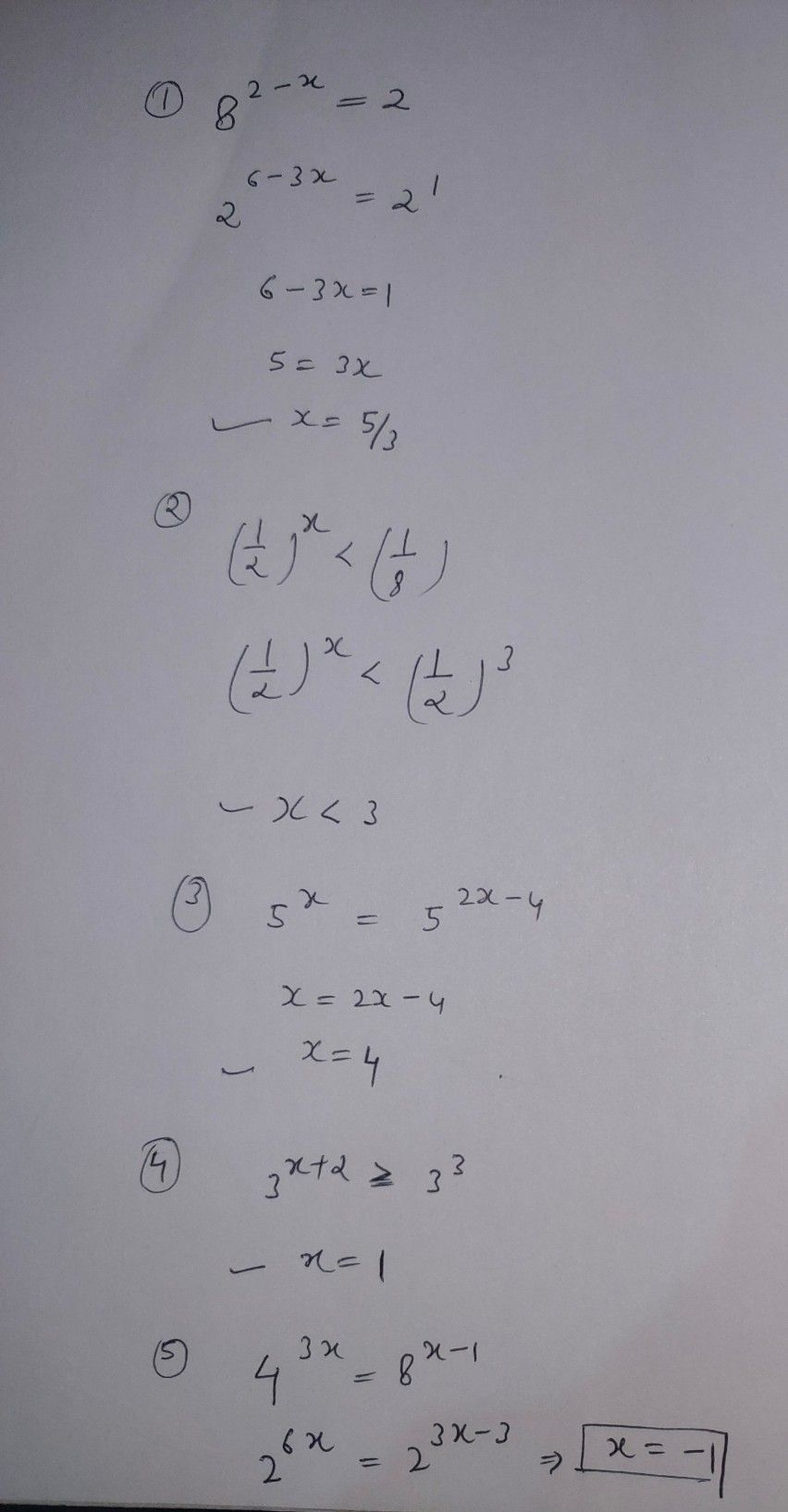Symbol
Problemivity $1:$ Find the Missin e for the values of $x$ for $1$ $8^{2-x}=2$ $2$ $\left(\dfrac {1} {2}\right)^{x}$ $<\dfrac {1} {8}$ $3$ $5^{x}=25^{x-2}$ $y$ $3^{x+2}\geq 27$ $5$ | $4^{3x}=8^{x-1}$ y $2$ Use graphing p be your graph briefly $-2\times 2$
10th-13th grade
Algebra
Search count: 113
SolutionQanda teacher - AvailableHi dear, thanks for asking question in preferred match you can discuss your daily homework with me I'll solve them immediately
Please give 3000 coins as thank you gift this will not reduce your coins
Happy Learning ??